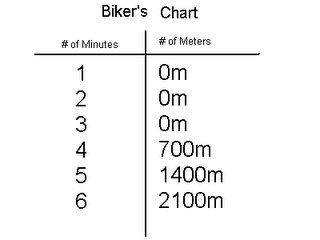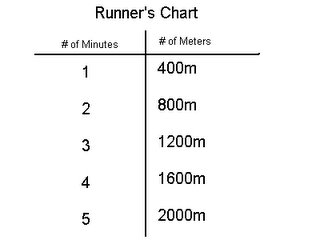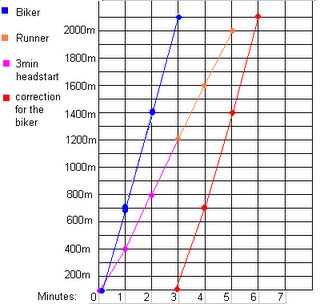## Monday, May 29, 2006

### Kim's growing Post for May 29

Question 1.
What is the difference between an algebraic expression and an equation (hint:one contains this and the other other doesn't?)

The difference bewteen an algebraic expression and an equation is that the algebraic expression has not given the value of the variable and the equation has already given us the value of the variable. (example:)
expression: 10 + 5n = the answer
equation: 10 + 5(3) =
45

Question 2.
What is a variable and why do we use one in algebra.

A variable represents the unknown number. We use a variable in algebra because the main thing about algebra is to find the unknown answer and since the variable is the unknown number, we have to solve the variable. (And if you have solved the variable, it makes it a whole lot easier to solve the equation.)

Question 3.
Solve for N.

I need to solve how much the triangular equals to solve the question. After that, I need to solve how much the 2 N's equal to.
Solving for first scale: Since a square means 2 circles and a circle means 1, and the left side of the scale in this diagram has 2 squares, that means 4 circles or 4 in all. So I take 4 circles away from the right hand of the scale, I will end up having only 5 circles left and a triangle. So that means a triangle is equal to 5 circles.
Solving for second scale: Since I have already solved the value of the triangle and I know what the value of the circle and the square is, I will be able to solve the value of the 2 N's easily. First I would have to add all the squares and triangles on the right hand of the scale, and it would come out of a total of 22 circles or 22 (since a triangle represents 5 circles and a square represents 2 circles.) Now I will have to divide the number 22 in half (because there are 2 Ns) and I will get 11. So the value of each N will be 11. The total value of both Ns is 22 circles.

Question 4.
Solve the following equations. Show all of the steps that are needed.

a) 3n + 4n + 7 = 2n + 12
3n + 4n - 2n = 12 - 7
5n = 5
n = 5/5 = 1
n = 1

b) 8n - (4 + 9) = 11
8n - 13 = 11
8n = 11 + 13 = 24
8n = 24
n = 24/8 = 3
n = 3

c) (8-3)n + 7n + 8 = 4n + 40
5n + 7n + 8 = 4n + 40
5n + 7n - 4n = 40 - 8
8n = 32
n = 32/8 = 4
n = 4

Question 5. For this question you need to create a T-chart and a graph to plot your data from this question onto.
You are having a race against your friend, except you are on foot and he is on his bike. You both know that if you are on bike you will be faster so your friend gives you a head start of 3 minutes. If you can run 400m per minute, and your friend can bike 700m per minute, who will be the first one to make it to the finish line 2000m away?The runner will come in first in 5 minutes and the biker will come in second in about 6 minutes.

At 5/30/2006 9:21 AM,amelia_ mal said...

Hey Kim! That was great work. You have put alot of effert on it. it's Amalia

At 5/30/2006 9:44 AM,kimbutt said...

Hey kim.Good job on your growing post.I like yours.

At 5/30/2006 3:33 PM,Fang_the_Barbarian said...

GREAT JOB!!!!!

At 6/01/2006 2:34 PM,Smiiliies said...

At 6/01/2006 3:54 PM,Mr. Reece said...

Only a few small additions that need to be made. First for question 1, you aren't given the variable in a equation, you still need to fin out what the variable's value is since it is the unknown, rather you are given a solution to the balance the expression.

For question three don't circles have a value?

Finally on your graph I think that the biker has started in the wrong place, as he/she doesn't move until minute 3 according to your T-chart.

Otherwise great job!

At 6/19/2006 2:33 PM,Mr. Reece said...

At 12/11/2017 11:42 PM,Love Kpop said...

Not all are true. Everyone has their own way of thinking but I think they have to reconsider. I like to argue for the most accurate results.
fivenightsatfreddysplay.com

At 1/19/2018 5:47 PM,learn to fly 2 said...

Thanks for a wonderful share. Your article has proved your hard work and experience you have got in this field. Brilliant .i love it reading.